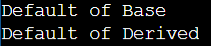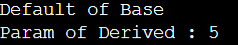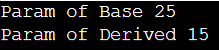# How C++ Constructors are Called in Inheritance

## How C++ Constructors are called in Inheritance with Examples

In this article, I am going to discuss How C++ Constructors are called in Inheritance with Examples. Please read our previous article where we discussed Inheritance in C++ with Examples. Constructor is a class member function with the same name as the class name. The main job of the constructor is to allocate memory for class objects. Constructor is automatically called when the object is created. It is very important to understand how constructors are called in inheritance.

##### How we can access Base class members using Derived Class Object in C++?

We know when we create an object, then automatically the constructor of the class is called and that constructor takes the responsibility to create and initialize the class members. Once we create the object, then we can access the data members and member functions of the class using the object.

In inheritance, we have Base/Parent/Superclass as well as Derived/Child/Subclass. If we create an object of the Base class, then the base class constructor is called and initializes the base class members, and then using the base class object, we can call the data members and member functions of the base class.

When we create an instance of the Derived class, then the Derived Class constructor is called and initializes the Derived Class members. But using the Derived class object we can access both the base class and derived class members. How?

How we can access the base class members using the derived class object? How the base class constructor is called? When the base class members are created?

Actually, when we create an object of the Derived class, the derived class constructor is called and initializes the derived class members. Also, the derived class constructor is either implicitly or explicitly called the Base class constructor and when the base class constructor is called, base class members are also created and initialized. This is the reason using the derived class object we can access both base class and derived class members.

##### How C++ Constructors are called in Inheritance?

Now let us see an example to understand how the C++ Constructors are called in inheritance. Here, we are taking a simple example. We are taking an example of class Base. In the base class we will not write anything, just write constructors as follows:

```class Base
{
public:
Base ()
{
cout << "Default of Base" << endl;
}
Base (int x)
{
cout << "Param of Base " << x << endl;
}
};```

This is our Base class which has two constructors. The first constructor is the default constructor that will print “Default of Base” when the object is created. The second constructor is parameterized constructor that will print “Param of Base” then it will print the value of x.

Now we will write a class called Derived that will be inheriting from the Base class. Inside the Derived class, we will not write any things, just defined the constructors as follows.

```class Derived : public Base
{
public:
Derived ()
{
cout << "Default of Derived" << endl;
}
Derived (int a)
{
cout << "Param of Derived : " << a << endl;
}
};```

This is our Derived class. This class is inherited from the Base class. It has two constructors. The first is a non-parameterized or default constructor that will print “Default of Derived” when called. The second is a parameterized constructor that will print “Param of Derived” then it will print the value of a.

So, we have two classes that are Base class with its default and parameterized constructor and the Derived class with its own default and parameterized constructor.

Now let us create an object of the Derived class and see how the constructors are executed. So, inside the main method, we will create an object of the Derived class as follows.

int main(){
Derived d;
}

Here we have created an object d of class Derived and not passing any argument means which constructor we are calling? We are trying to call the Derived() constructor. But we also know that along with the Derived class constructor, the parent class i.e. Base class constructor will also execute. But there are two constructors in the parent class which constructor will execute? Default constructor i.e. Base(). So, by default, the default constructor of the parent class will be executed.

##### Example: Executing the Parent Class Default Constructor automatically in C++
```#include <iostream>
using namespace std;
class Base
{
public:
Base ()
{
cout << "Default of Base" << endl;
}
Base (int x)
{
cout << "Param of Base " << x << endl;
}
};

class Derived : public Base
{
public:
Derived ()
{
cout << "Default of Derived" << endl;
}
Derived (int a)
{
cout << "Param of Derived" << a << endl;
}
};

int main()
{
Derived d;
}
```
###### Output:Let us see what happened here. First, it displayed, “Default of Base” and then it displayed “Default of Derived”. That means when you create an object of the Derived class then first the base class constructor will be executed then the Derived class constructor will be executed.

So, the point that you need to remember is whenever you are creating an object of a derived class then first the constructor of the base class will be executed and then the constructor of the derived class will be executed.

##### Which constructor of the parent class will be executed?

Always the default constructor of the parent class will be executed. Let us pass some value in object d as follows.

int main(){
Derived d (5);
}

Here we have passed 5 as a parameter in the constructor of the Derived class object. In this case, we have created an object of the Derived class by calling the parameterized constructor with a value of 5. But we know very well that the Derived class constructor will not execute first. The Base class constructor will execute. So which constructor will execute in the Base class? Again, the default constructor of the base will execute. So, first “Default of Base” will be printed on the screen. Then after that, it will come back and execute the Derived class parameterized constructor. “Param of Derived 5” will be printed. The complete example is given below.

```#include <iostream>
using namespace std;
class Base
{
public:
Base ()
{
cout << "Default of Base" << endl;
}
Base (int x)
{
cout << "Param of Base " << x << endl;
}
};

class Derived : public Base
{
public:
Derived ()
{
cout << "Default of Derived" << endl;
}
Derived (int a)
{
cout << "Param of Derived : " << a << endl;
}
};

int main()
{
Derived d(5);
}
```
###### Output:So still the default constructor of Base class and then parameterized constructor of Derived class has executed.

##### How to execute the Parameterized Constructor of Base class in Inheritance?

Now we want to call the parameterized constructor of the Base class when the object of the Derived classes is executed. So, for that, we should have a special constructor in the Derived class as follows which will call the base class parameterized constructor.

Derived(int x, int a) : Base(x){
cout << “Param of Derived ” << a;
}

Here we have written another parameterized constructor in the Derived class. This constructor is taking two integer type parameters that are x and a. Then we have written “: Base (x)”. So, here we are calling the parameterized constructor of the Base class with x as a parameter. Next, we have written a printing statement “Param of Derived” and then print the value of a. So, here from the Derived class constructor we are explicitly calling the parameterized constructor of the Base class. So let us write another statement inside the main function as follows:

int main(){
Derived d (25, 15);
}

Here we are giving two parameters in the constructor of the Derived object. Now, the parameterized constructor of the Derived class will be called which is taking two parameters.

This constructor will take 25 in x and 15 in a. Then the Derived class constructor will call Base(25). The Base class parameterized constructor will be called. So, in this way, we can call the base class parameterized constructor from the derived class constructor. The complete example code is given below.

```#include <iostream>
using namespace std;
class Base
{
public:
Base ()
{
cout << "Default of Base" << endl;
}
Base (int x)
{
cout << "Param of Base " << x << endl;
}
};

class Derived : public Base
{
public:
Derived ()
{
cout << "Default of Derived" << endl;
}
Derived (int a)
{
cout << "Param of Derived : " << a << endl;
}
Derived(int x, int a) : Base(x)
{
cout << "Param of Derived " << a;
}
};

int main()
{
Derived d(25, 15);
}
```
###### Output: